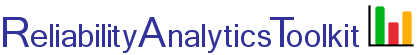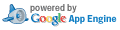# Weibull Analysis Background Information

The resulting analysis provides estimates for the Weibull shape parameter, β, and characteristic life, η. The reliability and hazard rate can be determined estimated at a specific point in time (x) using the derived β and η. Using these parameters, the Weibull distribution can then be used to model many different failure distributions. The two-parameter Weibull distribution probability density function, reliability function and hazard rate are given by: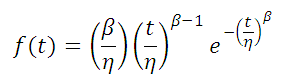Probability Density Function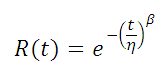Reliability Function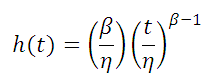Hazard Rate

A three-parameter Weibull Distribution also includes a location parameter, sometimes termed failure free life. For these cases, t in the above above equations is replaced by t-δ.

The characteristic life, η, is the point where 63.2% percent of the population will have failed. This is true regardless of the shape parameter (β) chosen. The following shape parameter characteristics are noted:

β = 1.0 : Exponential distribution, constant failure rate
β = 3.5 : Normal distribution (approximation)
β < 1.0 : Decreasing failure (hazard) rate
β > 1.0 : Increasing failure (hazard) rate

Median ranks are calculated using the following equation.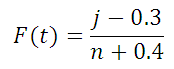Median Rank Equation

where:

j is the failure order
n is the sample size

Data that contains non-failed items is termed "censored," or "suspended" test data. For this type of data adjusted ranking, or ordered points, are calculated and used to calculate F(t), the cumulative fraction failed. This modified order is then used for j in the above median rank equation. The column labled Increment in the table is calculated using the following equation.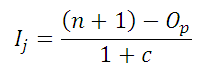Increment Equation

where:

Ij is the increment for point j.
n is the total number of records, both failures and censored items.
Op is the order of the previous point, or record.
c is the number of remaining points, including the current point.

The order of each point, Oj, is the order of the previous point, Op, plus Ij calculated according to the above equation. These calculations are shown for each point in the table columns labeled Increment and Order in the calculation results.

The calculation inputs are show units of "hours," but any life metric (cycles, years, miles, etc.) can be used as long as there is consistency among the inputs.

Toolkit Home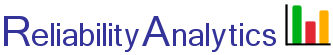reliabilityanalytics.com

### References:

1. MIL-HDBK-338, Electronic Reliability Design Handbook.
2. Nelson, Wayne, Applied Life Data Analysis
3. Dodson, Bryan, The Weibull Analysis Handbook.
4. Dodson, Bryan, The Weibull Analysis Handbook.
5. Abernethy, Robert, The Weibull Analysis Handbook.
6. Bazovsky, Igor, Reliability Theory and Practice.
7. O'Connor, Patrick, D. T., Practical Reliability Engineering.
8. http://en.wikipedia.org/wiki/Weibull_distribution
9. http://en.wikipedia.org/wiki/Maximum_likelihood
10. Weibull Distribution, NIST Engineering Statistics Handbook .
11. Khan Academy, Probability density functions for continuous random variables..
12. Barranger, Paul, See http://www.barringer1.com/wdbase.htm for a database of typical Weibull shape and characteristic life parameters.
13. http://reliawiki.org/index.php/Weibull_Distribution_Examples
14. Kececioglu, Dimitri, Reliability & Life Testing Handbook.